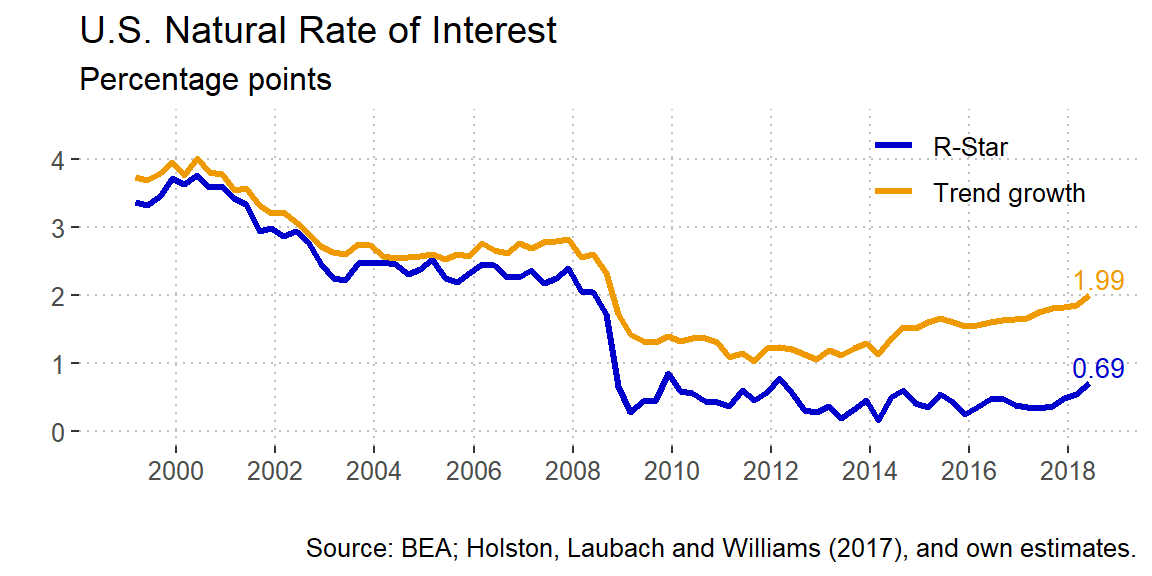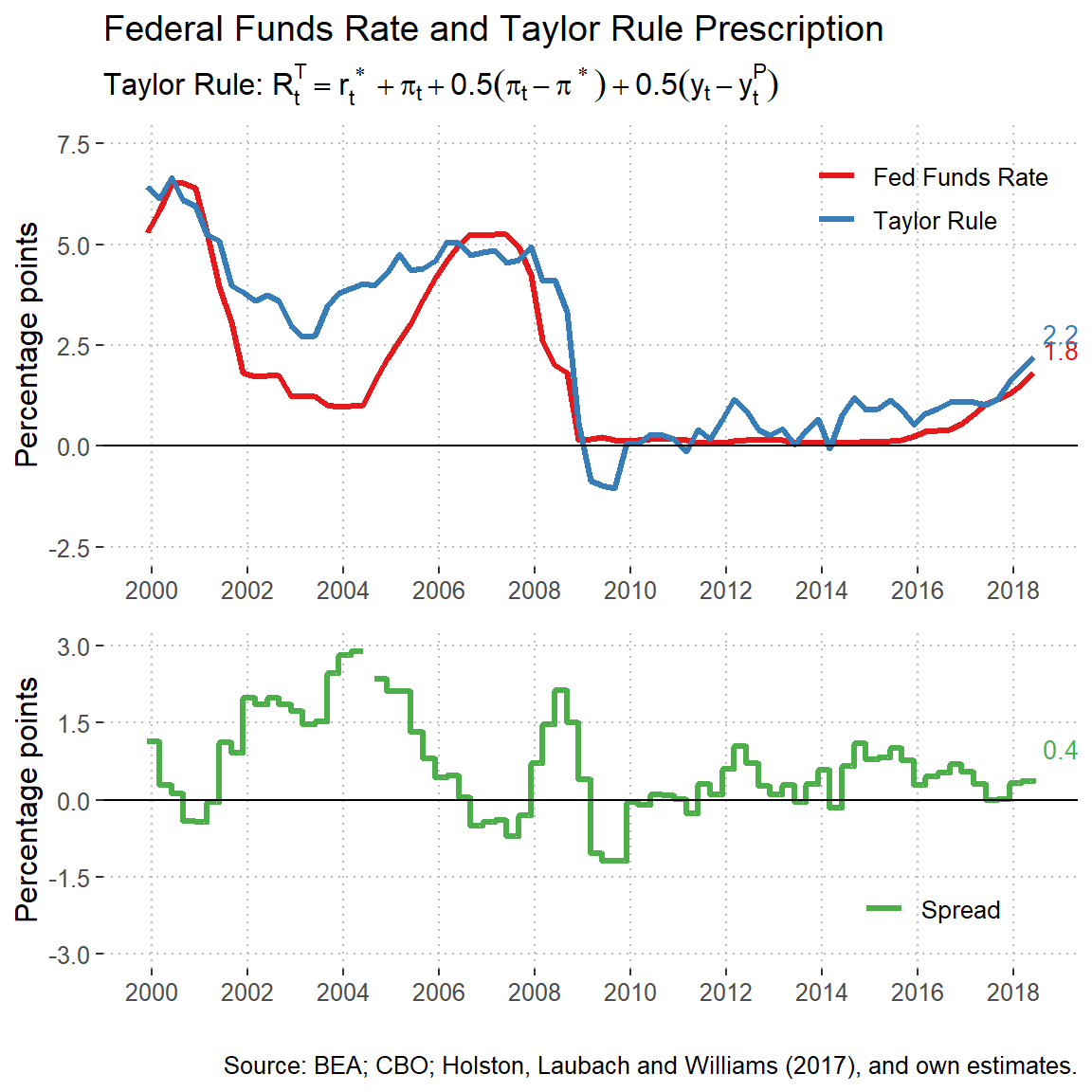# The Natural Interest Rate and the Taylor Rule Prescription

Economics
Author

Rodrigo Mariscal

Published

September 30, 2018

### Monetary Stance in the U.S.

##### The Natural Interest Rate (R-Star)

The natural interest rate or r-star is commonly defied as the long-term interest rate such that monetary policy is consistent sustained maximum employment and inflation at its longer-run objective.1 Also it’s sometimes defined as the interest rate that would preveil in a steady state where prices are fully flexible and inflation is zero (Woodford, 2003). However, the approach that I will take here is the one put forward by Holston, Lauback and Williams (2017) which is more an equilibrium concept that uses a time series approach.

It’s imporant to note that this $$r_{t}^{*}$$ variable is attached to slow-moving forces that hardly change except when there is a big disruption in the economy. As an example, these are the forces that can affect the natural interest rate:

• Changes in labor productivity that affect the amount of labor supplied or the return on investment
• Household’s desire to save or to realocate current consumption from the future
• Public debt or government consumption that affect the use of aggregate savings in the economy
• Capital flows, that is, the amount of financial resources coming from the rest of the world
• The risk premia that the savers would like to received in return for their borrowed assets### Monetary Policy Rules

Policy Rules Formula
Taylor Rule $$R_t^T = r_t^{*} + \pi_t + 0.5(\pi_t - \pi^{*}) + 0.5(y_t - y_t^P)$$
Inertial rule $$R_t^I = 0.85R_{t-1} + 0.15[r_t^{*} + \pi_t + 0.5(\pi_t - \pi^{*}) + (y_t - y_t^P)]$$
Balanced-approach rule $$R_t^{BA} = r_t^{*} + \pi_t + 0.5(\pi_t - \pi^{*}) + (y_t - y_t^P)$$
ELB-adjusted rule $$R_t^{ELB} = max\{ R_{t-1}^{BA} - Z_t, 0 \}$$
First-difference rule $$R_t^{FD} = R_{t-1} + 0.1(\pi_t - \pi^*) + 0.1(y_t - y_{t-4})$$

### Taylor Rule (inertial)

The Taylor Rule originally proposed by John B. Taylor is a prescription for the level of federal funds rate such that the monetary policy supports price stability and sustain GDP growth. The general formulation of the rule is the following:

$$R^{T}_{t} = \rho R^{T}_{t-1} + (1 - \rho) [ r^{*} + \bar{\pi}_t + \beta ( \pi_{t} - \bar{\pi}_t ) + \gamma x_{t} ],$$

where $$R^{T}_{t}$$ is the federal funds prescribed rate in quarter t, $$r_t^{*}$$ is the natural interest rate estimated above, $$\pi_{t}$$ is the four-quarter inflation rate, $$\bar{\pi}_t$$ is the central bank’s objective for inflation, and $$x_{t}$$ measures the percentage difference of GDP from its potential level or output gap. The $$\rho$$ parameter is a measure of persistence that smooths the series to make gradual adjustments in the policy rate, $$\beta$$ is the weight on the inflation gap and $$\gamma$$ the weight on the output gap.## Footnotes

1. Federal Reserve Board of Governors. 2018. ‘’Monetary Policy Report - February 2018’’, Federal Reserve Board, web site.↩︎